Test: Diode Circuits

# Test: Diode Circuits

Test Description

## 10 Questions MCQ Test Analog Electronics | Test: Diode Circuits

Test: Diode Circuits for Electrical Engineering (EE) 2023 is part of Analog Electronics preparation. The Test: Diode Circuits questions and answers have been prepared according to the Electrical Engineering (EE) exam syllabus.The Test: Diode Circuits MCQs are made for Electrical Engineering (EE) 2023 Exam. Find important definitions, questions, notes, meanings, examples, exercises, MCQs and online tests for Test: Diode Circuits below.
Solutions of Test: Diode Circuits questions in English are available as part of our Analog Electronics for Electrical Engineering (EE) & Test: Diode Circuits solutions in Hindi for Analog Electronics course. Download more important topics, notes, lectures and mock test series for Electrical Engineering (EE) Exam by signing up for free. Attempt Test: Diode Circuits | 10 questions in 10 minutes | Mock test for Electrical Engineering (EE) preparation | Free important questions MCQ to study Analog Electronics for Electrical Engineering (EE) Exam | Download free PDF with solutions
 1 Crore+ students have signed up on EduRev. Have you?
Test: Diode Circuits - Question 1

### For the given circuits and input waveform determine the output waveform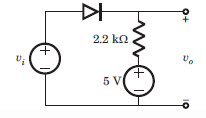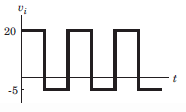Detailed Solution for Test: Diode Circuits - Question 1

Diode is off when Vi < 5

When Vi > 5, Vo = 5V

Test: Diode Circuits - Question 2

### For the given circuits and input waveform determine the output waveform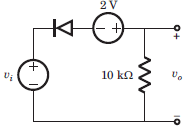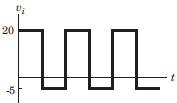Detailed Solution for Test: Diode Circuits - Question 2

Diode is off when Vi + 2 > 0.

Test: Diode Circuits - Question 3

### For the given circuits and input waveform determine the output waveform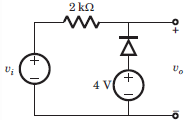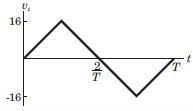Detailed Solution for Test: Diode Circuits - Question 3

For V1 < 4 the diode is on otherwise its off.

Test: Diode Circuits - Question 4

For the given circuits and input waveform determine the output waveform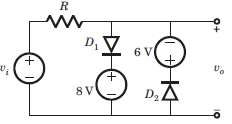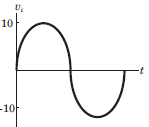Detailed Solution for Test: Diode Circuits - Question 4

During +ve half cycle when Vi < 8 both the diodes are off. For V1 > 8, D1 is on. Similarly for the negative half cycle.

Test: Diode Circuits - Question 5

The maximum load current that can be drawn is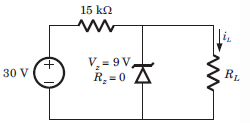Detailed Solution for Test: Diode Circuits - Question 5

At regulated poer supply, Is = 30-9/15k or 1.4 mA. The load current will remain less than this current.

Test: Diode Circuits - Question 6

For the circuit shown diode cutting voltage is Vin = 0. The ripple voltage is to be no more than vrip = 4 V. The minimum load resistance, that can be connected to the output is (in kilo ohm)​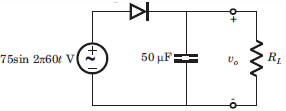Detailed Solution for Test: Diode Circuits - Question 6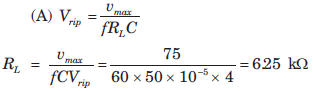Test: Diode Circuits - Question 7

The circuit inside the box in fig. P3.1.31. contains only resistor and diodes. The terminal voltage vo isconnected to some point in the circuit inside the box. The largest and smallest possible value of vo most nearly to is respectively​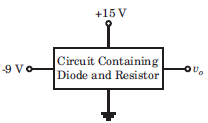Detailed Solution for Test: Diode Circuits - Question 7

The output voltage cannot exceed the positive power supply voltage and cannot be lower than the negative power supply voltage.

Test: Diode Circuits - Question 8

The Q-point of the zener diode in the circuit shown below is​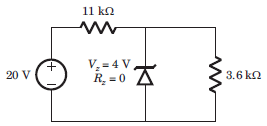Detailed Solution for Test: Diode Circuits - Question 8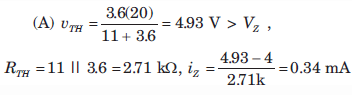Test: Diode Circuits - Question 9

A 2-port network is driven by a source Vs = 100 V in series with 5 ohm, and terminated in a 25 ohm resistor. The impedance parameters are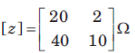The Thevenin equivalent circuit presented to the 25 ohm resistor is

Detailed Solution for Test: Diode Circuits - Question 9

100 = 25I1 + 2I2, V2 = 40I1 + 10I2
Also V2 = 160I1 + 6.8I2
using above equations we get, Vth = 160 V and Rth = 6.8 ohm.

Test: Diode Circuits - Question 10

Find V1 and V2.​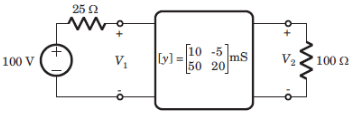Detailed Solution for Test: Diode Circuits - Question 10

800 = 10V1 – V2 and 3V2 = 5V1 = 0.

## Analog Electronics

11 videos|57 docs|108 tests
Information about Test: Diode Circuits Page
In this test you can find the Exam questions for Test: Diode Circuits solved & explained in the simplest way possible. Besides giving Questions and answers for Test: Diode Circuits, EduRev gives you an ample number of Online tests for practice

## Analog Electronics

11 videos|57 docs|108 tests(Scan QR code)